##用户名 UID Email 自动登录 找回密码 密码 立即注册
 注册新账号 忘记密码?

#勋章中心

•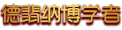上古百科贡献者

•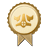百科之魂

•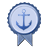百科开拓者

•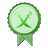百科引导者

•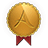庭院贡献者

•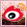[微博粉丝专属]

•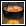厨艺达人

•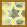原大陆之星

•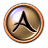上古印记Ⅰ

•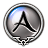上古印记Ⅱ

•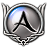上古印记Ⅲ

•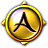上古印记Ⅳ

•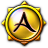上古印记Ⅴ

•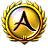上古印记Ⅵ

•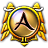上古印Ⅶ

•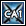[世界的雕琢]

•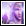[神启]

•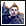[圣职]

•[航海季]

•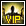[永久VIP]

•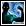[原驻民]

•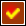[认证居民]

•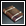[学者]

•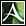[古灰]

•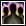[元老院]

•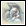[远征军]

•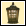[明灯]

•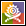[哈里兰]

•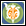[精灵]

•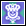[诺亚]

•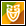[兽灵]

•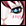[捏捏捏]

•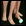[第一次]

•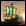[游记]

•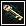[贡献者]

•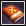[圣传]

•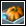劳动最光荣

•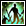[后花园]

•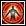[原创达人]

•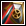[守护者]

•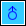[上古GG]

•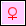[上古MM]

•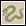[蛇年勋章]

•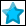哈尔法之星

•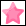哈尔法之星

•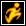[逃狱者]

•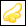[最受欢迎版主]

•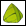[粽子]

•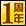[1周年]

•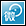[微圈子]

•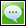[微信]

•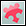[拼拼拼拼]

•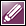[AGE记者团]

•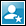[巡查]

•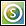[搜搜]

•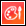[表情达人]

•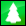[圣诞树]

•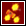[春节]

•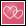[情人节]

•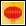[元旦]

•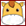[马年勋章]

•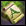[真情]

•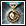[活动执行者]

•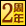[2周年]

•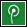微圈子纪念勋章

•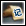航海日志纪念

•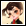定制脸型

•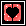德翡纳探大险家

•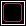德翡纳卓越贡献者

•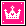德翡纳终身成就

•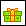[天黑黑1]

•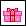[天黑黑2]

•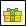[天黑黑3]

•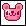[萌宠]

•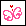[女人心]

•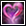[幸运]

•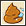[糗事专属]

•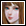[庭院娘]

•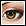[小眼睛大发现]

•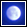[朦胧月色]

•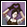[我爱我师]

•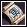[集集乐]

•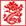[龙抬头]

•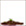[植树节]

•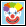[愚人节]

•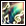[音乐达人]

•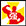[新古灰]

•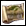[家园建设纪念]

•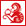猴年大吉

## 勋章

Archiver|手机版|《上古世纪》官方论坛 古灰们的家 |SuperBBS

GMT+8, 2021-1-23 07:26 , Processed in 0.159824 second(s), 10 queries .